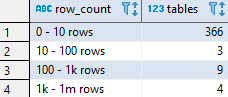## Number of tables by the number of rows in Redshift

Article for:

If you want to get an overview on how many rows tables in your database hold one way is to count them by row intervals. This query returns number of tables by the number of their rows grouped into predefined intervals.

## Query

``````select row_count,
count(table_name) as tables
from (
select tab.table_name,
tab.table_schema,
case when tinf.tbl_rows  > 1000000000 then '1b rows and more'
when tinf.tbl_rows  > 1000000 then '1m - 1b rows'
when tinf.tbl_rows  > 1000 then '1k - 1m rows'
when tinf.tbl_rows  > 100 then '100 - 1k rows'
when tinf.tbl_rows  > 10 then '10 - 100 rows'
else  '0 - 10 rows' end as row_count,
tinf.tbl_rows as sort
from svv_tables tab
left join svv_table_info tinf
on tab.table_schema = tinf.schema
and tab.table_name = tinf.table
and tab.table_schema not in ('pg_catalog', 'information_schema')
) as tables_rows_interval
group by row_count
order by max(sort);
``````

## Columns

• row_count - predefined row count intervals:
• 0 - 10 rows
• 10 - 100 rows
• 100 - 1k rows
• 1k - 1m rows
• 1m - 1b rows
• 1b rows and more
• tables - number of tables whose row count falls in that interval

## Rows

• One row: represents one interval
• Scope of rows: all row count intervals that appear in the database
• Ordered by: from the smallest table row count to the largest

## Sample results